# Stability analysis of differential scheme for dynamic equations of mooring cable system

## Ruizhi Shi1, Suxia Zhang2, Xijun Liu3, Fujiang Cui4, Anqi Zhou5

1, 2, 3, 4, 5Department of Mechanics, School of Mechanical Engineering, Tianjin University, Tianjin, 300354, P. R. China

1, 2, 3, 4, 5Tianjin Key Laboratory of Nonlinear Dynamics and Chaos Control, Tianjin, 300354, P. R. China

2Corresponding author

Vibroengineering PROCEDIA, Vol. 20, 2018, p. 267-272. https://doi.org/10.21595/vp.2018.20282
Received 4 October 2018; accepted 9 October 2018; published 19 October 2018

34th International Conference on Vibroengineering in Shanghai, China, October 19-21st, 2018

Copyright © 2018 Ruizhi Shi, et al. This is an open access article distributed under the Creative Commons Attribution License, which permits unrestricted use, distribution, and reproduction in any medium, provided the original work is properly cited.
Views 44
CrossRef Citations 0
Abstract.

The mooring cable system in plane motion can be modeled as two coupled partial differential equations, which can be numerical solved by finite difference method directly. The difference scheme is analyzed, and parameters selection for time-marching of displacement and velocity are deduced. The stability condition of the scheme is analyzed through Fourier series method, and parameters range which match stable scheme is given. Then, the parameters range is verified by a numerical example.

Keywords: mooring cable system, finite difference method, difference scheme, stability condition.

#### 1. Introduction

Cable structure is widely used in engineering because of its light weight and good flexibility, and mooring cable system is one of them. It is necessary to analyze the dynamic characteristics and tension of the cable to ensure engineering safety.

In order to accurately analyze the characteristics of cable structure, a large number of scholars have studied a lot of cable models and calculation methods. In the 1980s, Irvine  systematically summarized the previous studies on the static and dynamic state of different cable structures. It had ignored nonlinear factors in previous studies. Later, when considering the great influence of nonlinear factors on the system motion, scholars gradually take the nonlinear factors in the study of dynamic cable models. Perkins et al. [2-4] derived the three-dimensional dynamic equation of the fixed elastic cable through Hamilton principle, and studied the motion along the tangential, normal and auxiliary normal direction of the cable respectively; Then based on this model, the resonance response of elastic cable in steady flow is studied; Later, a theoretical model for the vibration of floating body with cable was established. According to this model, Zhang Suxia et al. [5, 6] studied the tension characteristic and impact tension under taut-slack condition. This model for characterize the spatial dynamical motion of elastic cables is still used today.

When solving the coupled system of partial differential equations, the decoupling solution can be obtained by Galerkin method; Meanwhile, it also can be solved directly by finite element or finite difference method , and the difference scheme used in the finite difference method must be stable. Therefore, in this paper, the stability analysis of the difference scheme used to solve the dynamic equations of the mooring cable system is carried out, which in order to obtain the condition of the absolute stability of the scheme, and parameters of the time- marching function are determined.

#### 2. Model

Dynamic equations of mooring cable system in plane can be written as :

(1)

where ${U}_{1}$ and ${U}_{2}$ represent tangential and normal displacement of the cable from static equilibrium respectively; $s$ is the arc length coordinate of the unstretched cable; $\rho$ is cable’s density, and ${\rho }_{w}$ is fluid's density; $A$ is the cross-sectional area; $P$ is the pretension; $E$ is the elastic modulus; $\kappa$ is the curvature of the cable from static equilibrium; $\epsilon$ is the dynamic Lagrangian strain defined as Eq. (2); $g$ is the gravitational acceleration; ${l}_{t}=\mathrm{s}\mathrm{i}\mathrm{n}\theta$ and ${l}_{n}=\mathrm{c}\mathrm{o}\mathrm{s}\theta$ are direction cosines, and $\theta$ is the angle between tangential direction of the cable equilibrium and horizontal direction based on absolute coordinates; ${F}_{1}$ and ${F}_{2}$ are tangential and normal restoring forces of the cable in motion, which can be approximated by Morrison formula as Eq. (3):

(2)
$\epsilon =\frac{\partial {U}_{1}}{\partial s}-\kappa {U}_{2}+\frac{1}{2}\left[{\left(\frac{\partial {U}_{1}}{\partial s}-\kappa {U}_{2}\right)}^{2}+{\left(\frac{\partial {U}_{2}}{\partial s}+\kappa {U}_{1}\right)}^{2}\right],$
(3)
$\left\{\begin{array}{l}{F}_{1}={C}_{at}{\rho }_{w}\frac{\pi {d}^{2}}{4}{\stackrel{¨}{U}}_{1}+{C}_{dt}\frac{{\rho }_{w}d}{2}\left|{\stackrel{˙}{U}}_{1}\right|{\stackrel{˙}{U}}_{1},\\ {F}_{2}={C}_{an}{\rho }_{w}\frac{\pi {d}^{2}}{4}{\stackrel{¨}{U}}_{2}+{C}_{dn}\frac{{\rho }_{w}d}{2}\left|{\stackrel{˙}{U}}_{2}\right|{\stackrel{˙}{U}}_{2},\end{array}\right\$

where ${C}_{at}$, ${C}_{dt}$, ${C}_{an}$ and ${C}_{dn}$ are tangential and normal drag coefficients; $d$ is the effective diameter of cable.

#### 3. Numerical solution by finite difference method

The model is a problem of partial differential equations, which can be numerical solved by finite difference method.

#### 3.1. Difference scheme and coefficient determination

The spatial differentiation can be obtained in reference . Fourth-order formulas for first and second derivatives are expressed as Eq. (4), (5):

(4)
$\frac{\partial {u}_{j}^{k}}{\partial s}=\frac{1}{24\mathrm{\Delta }s}\left[\begin{array}{ccccc}-50& 96& -72& 32& -6\\ -6& -20& 36& -12& 2\\ 2& -16& 0& 16& -2\\ ⋮& ⋮& ⋮& ⋮& ⋮\\ 2& -16& 0& 16& -2\\ -2& 12& -36& 20& 6\\ 6& -32& 72& -96& 50\end{array}\right]\left[\begin{array}{c}{u}_{j-2}^{k}\\ {u}_{j-1}^{k}\\ {u}_{j}^{k}\\ {u}_{j+1}^{k}\\ {u}_{j+2}^{k}\end{array}\right]+O\left(\mathrm{\Delta }{s}^{4}\right),$
(5)
$\frac{{\partial }^{2}{u}_{j}^{k}}{\partial {s}^{2}}=\frac{1}{24\mathrm{\Delta }{s}^{2}}\left[\begin{array}{cccccc}90& -308& 428& -312& 122& -20\\ 20& -30& -8& 28& -12& 2\\ -2& 32& -60& 32& -2& \\ \ddots & \ddots & \ddots & \ddots & \ddots & \ddots \\ & -2& 32& -60& 32& -2\\ 2& -12& 28& -8& -30& 20\\ -20& 122& -312& 428& -308& 90\end{array}\right]\left[\begin{array}{c}{u}_{j-2}^{k}\\ {u}_{j-1}^{k}\\ {u}_{j}^{k}\\ {u}_{j+1}^{k}\\ {u}_{j+2}^{k}\end{array}\right]+O\left(\mathrm{\Delta }{s}^{4}\right),$

where $k$ is the time coordinate and $j$ is the spatial coordinate.

A time-marching algorithm for solution of the nodal displacement ${u}_{j}^{k}$ and velocity ${v}_{j}^{k}$ is expressed in reference  as Eq. (6), (7):

(6)
${u}_{j}^{k+1}={u}_{j}^{k}+{v}_{j}^{k}\mathrm{\Delta }t+\frac{1}{2}{\beta }_{1}\mathrm{\Delta }{t}^{2}\left({a}_{j}^{k}+{\beta }_{2}{a}_{j}^{k+1}\right),$
(7)
${v}_{j}^{k+1}={v}_{j}^{k}+\frac{1}{2}{\alpha }_{1}\mathrm{\Delta }t\left({a}_{j}^{k}+{\alpha }_{2}{a}_{j}^{k+1}\right).$

The nodal acceleration ${a}_{j}^{k+1}$ at $k+$ 1 time point can be obtained by dynamic equations of the mooring cable system, while expressions on the right hand side of the equation are at k time point. $\mathrm{\Delta }t$ is the time step; ${\alpha }_{1}$, ${\alpha }_{2}$, ${\beta }_{1}$ and ${\beta }_{2}$ are weighting parameters, and the selection condition can be proposed by using the method of undetermined coefficients on second-order backwards differencing in time as Eq. (8):

(8)
$\frac{{\partial }^{2}{u}^{k+1}}{\partial {t}^{2}}=\frac{\frac{\partial {u}^{k+1}}{\partial t}-\frac{\partial {u}^{k}}{\partial t}}{\mathrm{\Delta }t}+O\left(\mathrm{\Delta }t\right)=\frac{{u}^{k+1}-{u}^{k}}{\mathrm{\Delta }{t}^{2}}-\frac{{u}^{k}-{u}^{k-1}}{\mathrm{\Delta }{t}^{2}}+O\left(\mathrm{\Delta }{t}^{2}\right).$

Here is in the same spatial coordinate, so subscript can be omitted.

Substitute Eq. (6), (7) into Eq. (8) leads to Eq. (9):

(9)
$\frac{{\partial }^{2}{u}^{k+1}}{\partial {t}^{2}}=\frac{1}{2}{\beta }_{1}{\beta }_{2}{a}^{k+1}+\frac{1}{2}\left({\alpha }_{1}{\alpha }_{2}+{\beta }_{1}-{\beta }_{2}\right){a}^{k}+\frac{1}{2}\left({\alpha }_{1}-{\beta }_{1}\right){a}^{k-1}+O\left(\mathrm{\Delta }{t}^{2}\right).$

Due to $\frac{{\partial }^{2}{u}^{k+1}}{\partial {t}^{2}}={a}^{k+1}$, there are three conditions for selecting ${\alpha }_{1}$, ${\alpha }_{2}$, ${\beta }_{1}$ and ${\beta }_{2}$ as Eq. (10):

(10)
$\left\{\begin{array}{l}{\alpha }_{1}={\beta }_{1},\\ {\beta }_{1}{\beta }_{2}=2,\\ {\alpha }_{1}{\alpha }_{2}+{\beta }_{1}-{\beta }_{2}=0.\end{array}\right\$

#### 3.2. Stability analysis of difference scheme

By using Fourier series method (Von Neumann method) , the stability condition for the above scheme is analyzed.

The magnitude of $\mathrm{\Delta }s$ is associated with the length of the mooring cable, therefore non-dimensional method is carried out for Eq. (1), let:

where $L$ is the original length of the mooring cable; and $M$ is the cable’s mass. For Fourier series method is effective only in the linear system, so linearize the equations is necessary. Through the cable static equilibrium, a relation equation between the pretension and the gravity can be obtained; then simplified coefficients of restoring forces are substituted by $\xi$:

Finally, a non-dimensional linear difference scheme can be got:

(11)

Substitute Eq. (4), (5) into Eq. (11), then the discrete Fourier Transform is used as Eq. (12):

(12)
${\stackrel{^}{u}}^{k+1}\left(\zeta \right)=\frac{1}{\sqrt{2\pi }}{\sum }_{j=-\mathrm{\infty }}^{\mathrm{\infty }}{e}^{-ij\zeta }{\stackrel{^}{u}}_{j}^{k+1},$

and derive out Eq. (13) to express $\stackrel{^}{u}$ which increases with time changes:

(13)
$\left[\begin{array}{l}{\stackrel{^}{u}}_{1}^{k+1}\left(\zeta \right)\\ {\stackrel{^}{u}}_{2}^{k+1}\left(\zeta \right)\end{array}\right]=G\left[\begin{array}{l}{\stackrel{^}{u}}_{1}^{k}\left(\zeta \right)\\ {\stackrel{^}{u}}_{2}^{k}\left(\zeta \right)\end{array}\right],$

here, $G$ is a two dimensional growth matrix as:

$G=\left[\begin{array}{ll}a& b\\ c& d\end{array}\right],$

where:

(14)
$a=\frac{\mathrm{\Delta }{t}^{2}}{1-{\xi }_{1}}\left\{\frac{\stackrel{^}{E}+\stackrel{^}{P}}{24\mathrm{\Delta }{\stackrel{^}{s}}^{2}}\left[-8{\left(\mathrm{c}\mathrm{o}\mathrm{s}\zeta -4\right)}^{2}+72\right]+\frac{\partial \stackrel{^}{P}}{\partial \stackrel{^}{s}}\frac{1}{24\mathrm{\Delta }\stackrel{^}{s}}\left[-8\left(\mathrm{c}\mathrm{o}\mathrm{s}\zeta -4\right)\mathrm{s}\mathrm{i}\mathrm{n}\zeta \right]-{\stackrel{^}{\kappa }}^{2}\stackrel{^}{P}\right\},$
(15)
$b=\frac{\mathrm{\Delta }{t}^{2}}{1-{\xi }_{1}}\left\{-\frac{2\stackrel{^}{P}\stackrel{^}{\kappa }+\stackrel{^}{E}\stackrel{^}{\kappa }}{24\mathrm{\Delta }\stackrel{^}{s}}\left[-8\left(\mathrm{c}\mathrm{o}\mathrm{s}\zeta -4\right)\mathrm{s}\mathrm{i}\mathrm{n}\zeta \right]-\left(\stackrel{^}{P}\frac{\partial \stackrel{^}{\kappa }}{\partial \stackrel{^}{s}}+\stackrel{^}{E}\frac{\partial \stackrel{^}{\kappa }}{\partial \stackrel{^}{s}}-\stackrel{^}{\kappa }\frac{\partial \stackrel{^}{P}}{\partial \stackrel{^}{s}}\right)\right\},$
(16)
$c=\frac{\mathrm{\Delta }{t}^{2}}{1-{\xi }_{2}}\left\{\frac{2\stackrel{^}{P}\stackrel{^}{\kappa }+\stackrel{^}{E}\stackrel{^}{\kappa }}{24\mathrm{\Delta }\stackrel{^}{s}}\left[-8\left(\mathrm{c}\mathrm{o}\mathrm{s}\zeta -4\right)\mathrm{s}\mathrm{i}\mathrm{n}\zeta \right]+\stackrel{^}{P}\frac{\partial \stackrel{^}{\kappa }}{\partial \stackrel{^}{s}}+\stackrel{^}{\kappa }\frac{\partial \stackrel{^}{P}}{\partial \stackrel{^}{s}}\right\},$
(17)
$d=\frac{\mathrm{\Delta }{t}^{2}}{1-{\xi }_{2}}\left\{\frac{\stackrel{^}{P}}{24\mathrm{\Delta }{\stackrel{^}{s}}^{2}}\left[-8{\left(\mathrm{c}\mathrm{o}\mathrm{s}\zeta -4\right)}^{2}+72\right]+\frac{\partial \stackrel{^}{P}}{\partial \stackrel{^}{s}}\frac{1}{24\mathrm{\Delta }\stackrel{^}{s}}\left[-8\left(\mathrm{c}\mathrm{o}\mathrm{s}\zeta -4\right)\mathrm{s}\mathrm{i}\mathrm{n}\zeta \right]-{\stackrel{^}{\kappa }}^{2}\left(\stackrel{^}{P}+\stackrel{^}{E}\right)\right\}.$

The eigenvalue equation is $\left(\lambda -a\right)\left(\lambda -d\right)-bc=$ 0, also can be written as ${\lambda }^{2}-\left(a+d\right)\lambda +ad-bc=$ 0. For the difference scheme as Eq. (11) is absolutely stable, the eigenvalue must satisfy the following condition:

(18)
$\left|\lambda \right|\le 1.$

This inequality can be separated two sets, one is:

(19)
${\left(a-d\right)}^{2}+4bc<0\cap \left|ad-bc\right|\le 1,$

and the other one is:

(20)
${\left(a-d\right)}^{2}+4bc\ge 0\cap a+d-ad+bc\le 1\cap a+d+ad-bc\ge -1.$

The difference scheme as Eq. (11) will be absolutely stable, if $\mathrm{\Delta }\stackrel{^}{t}$ and $\mathrm{\Delta }\stackrel{^}{s}$ satisfy either of Eq. (19), (20).

#### 4. Numerical example

According to reference , structure parameters and environmental parameters of the mooring cable are taken as Table 1. The cable motion belongs to forced vibration, and there supposes a sine displacement excitation on the upper point of the cable.

Substitute parameters into Eqs. (14)-(17) and make judgments about Eqs. (19), (20).

The results show that ${\left(a-d\right)}^{2}+4bc\ge$ 0 is always tenable, so $\mathrm{\Delta }\stackrel{^}{t}$ and $\mathrm{\Delta }\stackrel{^}{s}$ which match condition Eq. (19) are empty set. $\mathrm{\Delta }\stackrel{^}{t}$ and $\mathrm{\Delta }\stackrel{^}{s}$ which match condition Eq. (20) are shown in Fig. 1. Values range of $\mathrm{\Delta }\stackrel{^}{t}$ and $\mathrm{\Delta }\stackrel{^}{s}$ are from 0 to 1. $\mathrm{\Delta }\stackrel{^}{s}$ labels horizontal axis of the figure, and $\mathrm{\Delta }\stackrel{^}{t}$ marks the vertical axis. The difference scheme will be absolutely stable, if $\mathrm{\Delta }\stackrel{^}{t}$ and $\mathrm{\Delta }\stackrel{^}{s}$ are in the red area. The three black lines along the border of red area are condition Eq. (20) when the two sides are equal. If $\mathrm{\Delta }\stackrel{^}{t}$ and $\mathrm{\Delta }\stackrel{^}{s}$ are out of the red area, the result may be divergent.

Table 1. Structure parameters and environmental parameters of the mooring cable

 Parameter Data Parameter Data Original length $L$ (m) 1400 Fluid’s density ${\rho }_{w}$ (kg⋅m-3) 1000 Effective diameter $d$ (m) 0.12 Tangential drag coefficients ${C}_{at}$ 1 Linear density $\rho A$ (kg⋅m-1) 70.37 Tangential drag coefficients ${C}_{dt}$ 0.05 Cross-sectional area $A$ (m2) 0.0113 Normal drag coefficients ${C}_{an}$ 1 Elastic modulus $EA$ (kN) 136064 Normal drag coefficients ${C}_{dn}$ 1.2 Horizontal pretension at upper point ${P}_{0}$ (N) 8.5985×105 Vertical pretension at upper point $V$ (N) 1.29574×106

Fig. 1.$\mathrm{\Delta }\stackrel{^}{t}$ and $\mathrm{\Delta }\stackrel{^}{s}$ which match condition Eq. (20)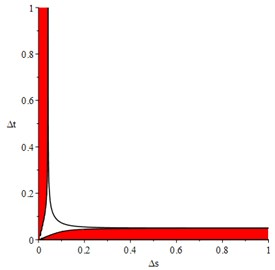Extract two sets of data, respectively in and out of the red area, for numerical solution of dynamic equations of mooring cable system. The results are shown in Fig. 2.

The time histories of tangential and normal displacement at 700 m point are shown in Fig. 2(a), (b). In these results, $\mathrm{\Delta }\stackrel{^}{t}=$ 0.001 and $\mathrm{\Delta }\stackrel{^}{s}=$ 1/280 are selected form the stable area, and we can get stable numerical solution. When $\mathrm{\Delta }\stackrel{^}{t}=$ 0.01 and $\mathrm{\Delta }\stackrel{^}{s}=$ 1/280, the results are divergent shown as Fig. 2(c), (d). That means the value selection must from the stable area, then convergent results can be got.

Furthermore, the numerical solution is more accurate if $\mathrm{\Delta }\stackrel{^}{t}$ and $\mathrm{\Delta }\stackrel{^}{s}$ are smaller. This difference scheme is absolutely stable only in linear system or some weak nonlinear system, so it’s better to solve nonlinear stability without linearization if nonlinear terms have strong effect of results.

Fig. 2. Numerical solution of dynamic equations of mooring cable system at $\mathrm{\Delta }\stackrel{^}{s}=$ 1/280: a) tangential displacement at 700 m point $\mathrm{\Delta }\stackrel{^}{t}=$ 0.001, b) normal displacement at 700 m point $\mathrm{\Delta }\stackrel{^}{t}=$ 0.001, c) tangential displacement at 700 m point $\mathrm{\Delta }\stackrel{^}{t}=$ 0.01, d) normal displacement at 700 m point $\mathrm{\Delta }\stackrel{^}{t}=$ 0.01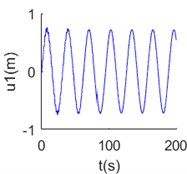a)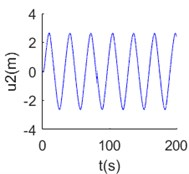b)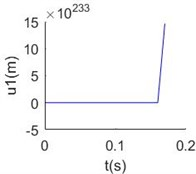c)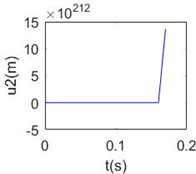d)

#### 5. Conclusions

The mooring cable system in plane motion is modeled as two coupled partial differential equations, which can be numerical solved by finite difference method without decoupling. This numerical method needs the difference scheme is stable and parameters selection is reasonable. Through the calculation and analysis, conclusions are obtained as follow:

1) Three conditions of time-marching parameters are derived, which used for displacement and velocity in the same space point, and second order accuracy can be satisfied to describe acceleration under these conditions;

2) The stability of the dimensionless linear difference scheme is analyzed by using Fourier series method, and conditions of the stability are obtained. Through a numerical example, the results show that the difference scheme can be stable under the linear system, and accurate numerical solutions can be obtained. However, in the nonlinear system, the influence of nonlinear terms should be considered.

#### Acknowledgements

The authors gratefully acknowledge the support of the National Natural Science Foundation of China (51479136), the Project of Tianjin Municipal Transportation Commission (2018B-33), the Project of Tianjin Natural Science Foundation (17JCYBJC18700).

1. Irvine Max H. Cable Structures. The MIT Press, Cambridge, Massachusetts, London, England, 1981. [Search CrossRef]
2. Perkins N. C., Mote C. D. Three-dimensional vibration of travelling elastic cables. Journal of Sound and Vibration, Vol. 114, Issue 2, 1987, p. 325-340. [Publisher]
3. Kim W. J., Perkins N. C. Two-dimensional vortex-induced vibration of cable suspensions. Journal of Fluid and Structures, Vol. 16, Issue 2, 2002, p. 229-245. [Publisher]
4. Kim W. J., Perkins N. C. Linear vibration characteristics of cable-buoy systems. Journal of Sound and Vibration, Vol. 252, Issue 3, 2002, p. 443-456. [Publisher]
5. Tang Yougang, Zhang Suxia, Zhang Haiyan Study on snap tension induced by taut-slack in a mooring system. Journal of Vibration and Shock, Vol. 27, Issue 4, 2008, p. 70-72. [Search CrossRef]
6. Zhang Suxia, Tang Yougang, Hou Shujun, Liu Xijun Characteristic analysis of stress wave in mooring line in taut-slack condition. Engineering Mechanics, Vol. 27, Issue 5, 2010, p. 53-59. [Search CrossRef]
7. Zhang Wensheng Finite Difference Methods for Partial Differential Equations in Science Computation. Higher Education Press, Beijing, China, 2006. [Search CrossRef]
8. Schiesser W. E. The Numerical Method of Lines Integration of Partial Differential Equations. Academic Press, San Diego, 1991. [Search CrossRef]
9. Nejad Mohammad Behbahani Wave Propagation in Elastic Cables with and Without Fluid Interaction. Mechanic Engineering in the University of Michigan, 1997, p. 79. [Search CrossRef]
10. Wang Xinggang Coupled Dynamic Analysis of Deepwater Floating Structure and the Mooring system. Shanghai Jiao Tong University Press, Shanghai, China, 2014, p. 36-37. [Search CrossRef]